Want to share your content on R-bloggers? click here if you have a blog, or here if you don't.Valentine’s day is a nice opportunity to post on hot and sexy topics… Well, it’s also an important day that I should not miss, probably as much as Saint Patrick’s my wife’s birthday. And as I mentioned last week (here), it is difficult to get the distribution of the age of marriage on the internet… So maybe we can build up a small model, to understand when do girls decide to get married…
Consider a young girl who knows that he will not meet thousands of men willing to marry her (actually, one can consider the opposite point of view, with young man who can find only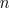girls willing to marry him, the problem can be assumed as symmetric, especially if I do not want to get feminist leagues on my back).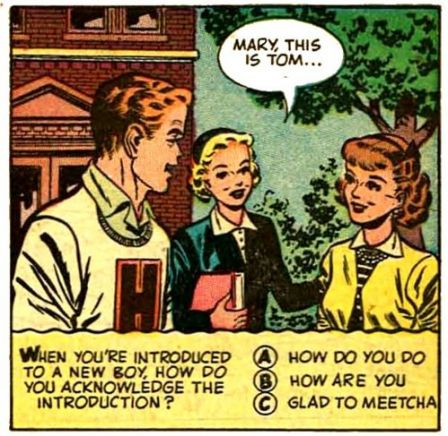Assume thatmen agree to marry her. Of course, among thosemen, our girl wants to marry the “best” one (assume that men can be ranked objectively). Of course, she cannot meet the “best” guy immediately, so men are met randomly, and after each “interview“, either she reject him (forever, we assume she cannot get back and admit she made a mistake), or agree to marry him. An important assumption is that rejected men cannot be recalled.

From a mathematical point of view, we need to find the optimal stopping time.
Here, the problem is slightly different compared with that one (with optimal time to get a bonus) or this one (with the optimal time to sit in a bar and have a beer). Here, we do not give “grades” to guy. The only thing that is observed is their relative ranks. Our girl cannot know if she’s meting the best of all men (out of), but she knows if this one is better than the ones she already met. From a mathematical point of view, at time, she knows the relative rank of(compared with the first), not his absolute rank. We also assume thatis known.

The optimal strategy is that she has to reject automatically the first(some kind of calibration period), and then, starting at time, she will marry the best over the ones she has already met.
So assume that our girl already met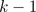guys, and decided to reject all of them. So now she’s trying to see if thecan be the optimal time to stop, and start looking seriously ….For an arbitrary cut-off, the probability that the best applicant will show up at some time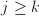is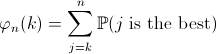i.e.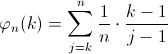The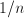term is because there is only one “best” guy, and theis the probability that he shows up at time(this can be visualized below)Thus, we can write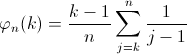i.e.Thus, since the minimum of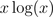is obtained when, which is the optimal time to stop (or here to start seeking).

Hence, the best strategy is to reject automatically the first=37% of the candidates (which is the maximum value of the function above), and then to select the first one (if possible) that is better than all previous candidates.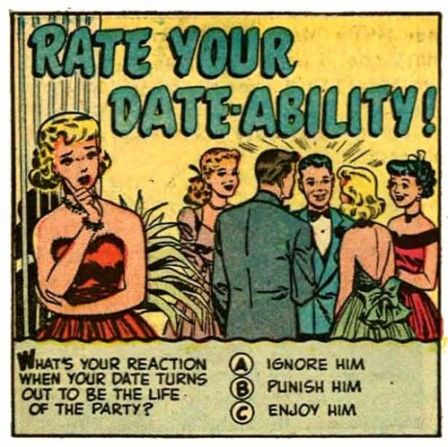Consider the following Monte Carlo procedure: assume that she rejects – automatically – the first(we consider a loop with all possible values for) and then gets married with the first one who is the best one she’s seen during the calibration period (or overall, which is the same),

```n=100
ns=1000000
MOY1=MOY2=rep(NA,n)
for(m in 2:(n-1)){
WHICH=rep(NA,ns); MARIAGE=rep(0,ns)
for(s in 1:ns){
Z=sample(1:n,size=n,replace=FALSE)
mx=max(Z[1:m])
STOP=FALSE
for(k in (m+1):n){
if((Z[k]>mx)&(STOP==FALSE)){
WHICH[s]=k
STOP=TRUE
MARIAGE[s]=1
}
}
}
HIS=WHICH[is.na(WHICH)==FALSE]
TH=table(HIS)
MOY1[m]=mean(HIS)
MOY2[m]=mean(HIS)*mean(MARIAGE)
THH=rep(NA,100)
THH[as.numeric(names(TH))]=as.numeric(TH)/ns
}```

If we run it over all possiblewe get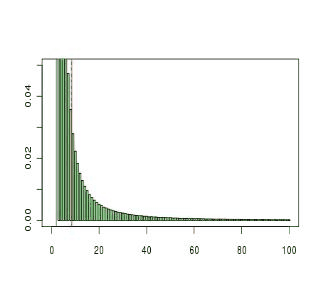The “distribution” (in green) can be seen as the probability to marry the guy of level, given that the firstwere rejected. The sum is not one since there is a non null probability to marry no one. Actually, the probability to get married is the following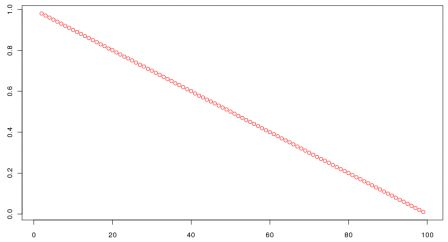The more she waits, the smaller the probability of getting married. But on the other hand, the more she waits, the “better” the husband…. On the graph below is plotted the rank of the guy she marries, if she gets married (it was actually the vertical plain line in red on the animation)So there is a trade-off. If not getting married gives a 0 satisfaction (lower than finally marrying anyone), and if marrying the guy with rankgives here satisfaction,we have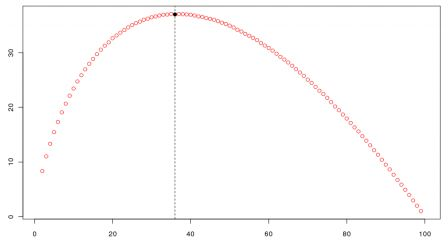(it was the vertical doted line in red on the animation). So it looks like it is optimal to test the first 35-38% men, and then to marry the best one she finds (if he is better than the best one she met during the “testing” procedure). So our previous analysis looks correct…
Now to go further, I have to admit that this model is known in academic literature as the secretary problem. In 1989, Thomas Ferguson wrote a nice paper in Statistical Science entitled who solved the secretary problem (here). Anthony Mucci published also an article in the Annals of Probability on possible extensions, in 1973 (here), or Thomas Lorenzen (there) in 1981. This problem is definitively an interesting one !